# 如何在 COMSOL Multiphysics®中使用规范固定？

2020年 4月 2日

### 固定规范的使用：通常没有必要

AC/DC 模块（COMSOL Multiphysics 的一个附加产品）中，有三个可求解矢量 \textbf{A} 的物理接口：

1. 磁场mf），它基于公式 \textbf{A}。，其中 \textbf{A} 是唯一的因变量。在稳态场中，如果不使用规范进行固定，则该解并不是唯一的；而在非稳态场中，由于感应区域的附加项具有数值意义，因此满足求解唯一性。
2. 磁场和电场mef），求解完全耦合的 \textbf{A} 场和 V 场。由于 规范变换，除非 \nabla\cdot\textbf{A}
指定，否则没有唯一解。
3. 旋转机械，磁rmm），公式 \textbf{A} 和 公式 V_m 的组合。公式 \textbf{A} 用于描述导电（存在电流）域，而公式 V_m 用于气隙和其他非导电域。这两个公式在它们共同的连接边界上耦合。由于 rmm 接口是混合公式，因此适用磁场mf）接口的规范固定特征。

mf, rmm

mfrmm

mef

mef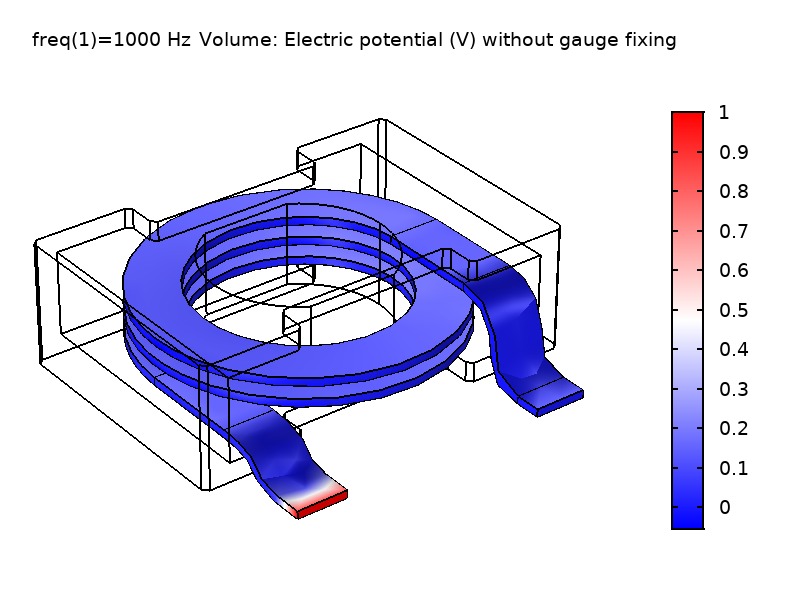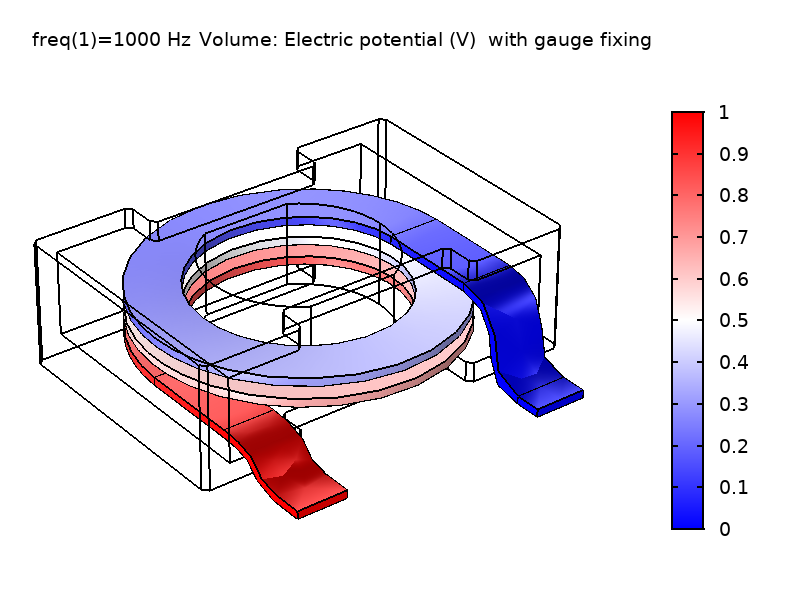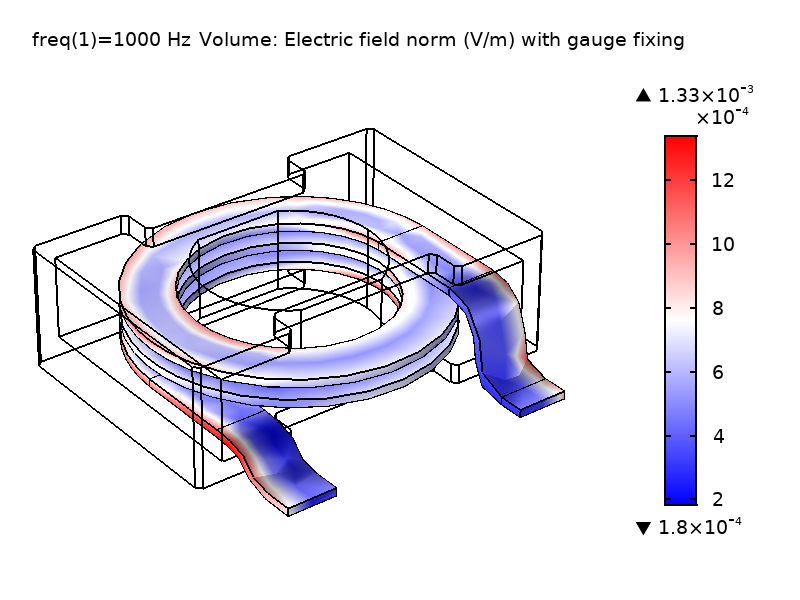### AC/DC 模块中的规范固定

mf mef rmm 接口中，有一个域条件称为磁矢势规范固定。除了在 2D 和 2D 轴对称中求解平面外磁矢势时，该功能均可使用。假定矢量势在平面外方向恒定，则可以轻松实现库仑规范\nabla\cdot\textbf{A} = 0

(1)

\nabla \times (\frac {1}{\mu}\nabla\times\textbf{A})=\textbf{J}_e+\nabla\psi

(2)

\nabla \cdot \textbf{A} = 0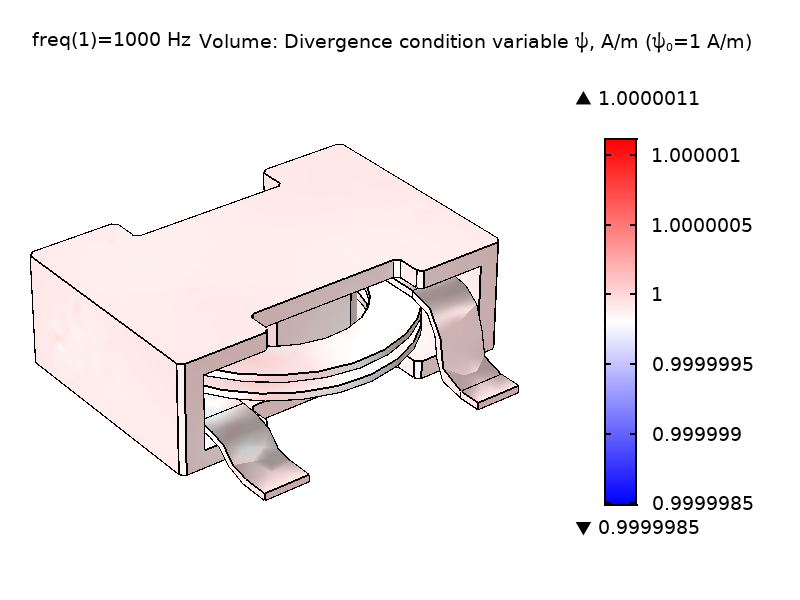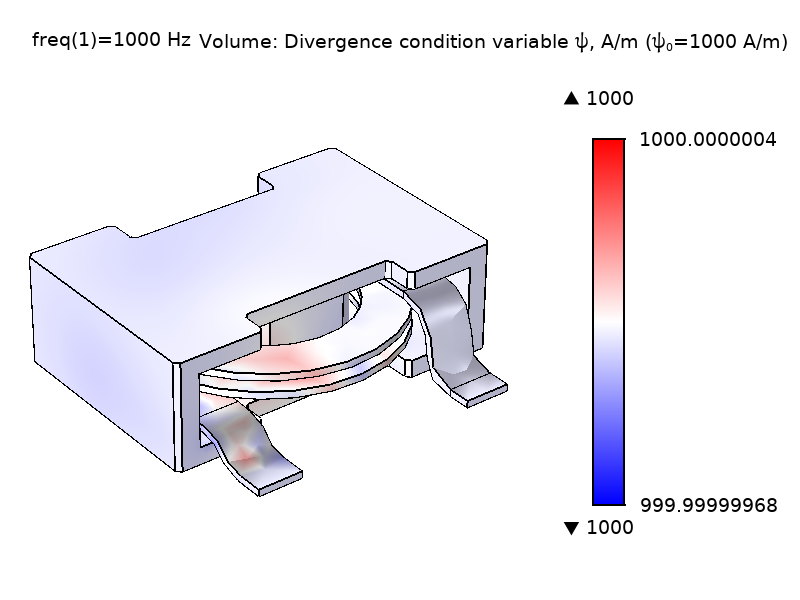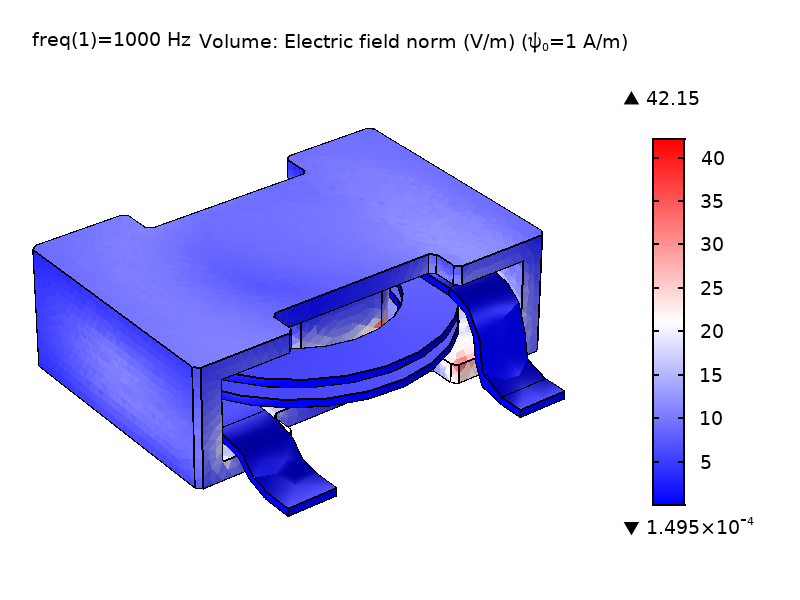### 增强电流转换：规范固定的另一个作用

(3)

\nabla\times(\frac{1}{\mu}\nabla\times\textbf{A})=\textbf{J}_e

(4)

\nabla\cdot(\nabla\times(\frac{1}{\mu}\nabla\times\textbf{A} ))=\nabla\cdot\textbf{J}

(5)

\sigma\frac {\partial \textbf{A}} {\partial t} + \nabla\times(\frac {1}{mu}
\nabla \times \textbf
{A} )= \textbf{J}

mf 稳态 亥姆霍兹线圈中的磁场

mef 频域 功率电感器的电感
rmm 瞬态 永磁电机三维模型